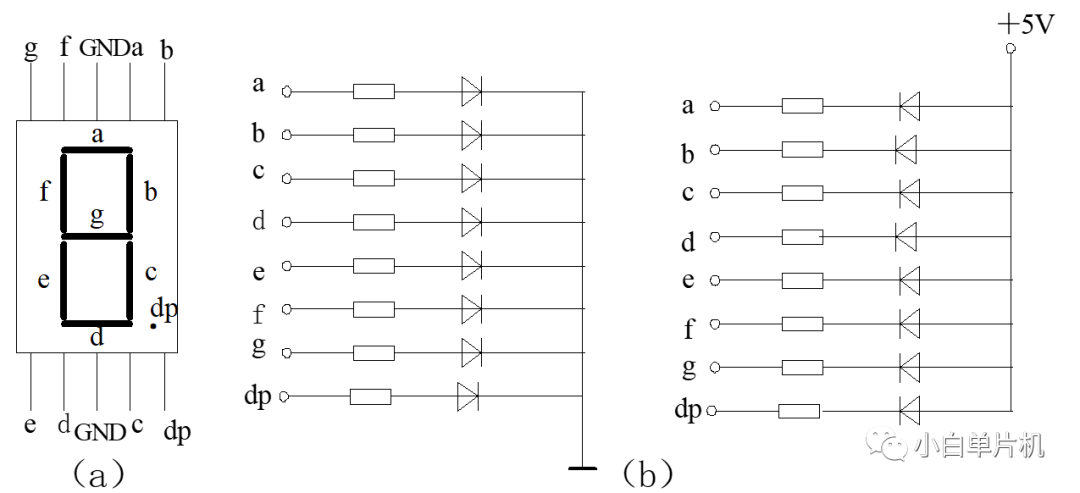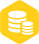# 单片机静态数码管的使用

### 描述b图的左侧，是共阴数码管，这是因为输出端口的另一侧统一接地；相反的，右侧的接法就是共阳了。

``````P0=0x39;
``````

``````void display()
{
u16 j=0;             //这里的u16 = unsigned int
for(j=0;j<8;j++)
{
switch(j)
{
case (0):aa=0;ab=0;ac=0;break;
case (1):aa=1;ab=0;ac=0;break;
case (2):aa=0;ab=1;ac=0;break;
case (3):aa=1;ab=1;ac=0;break;
case (4):aa=0;ab=0;ac=1;break;
case (5):aa=1;ab=0;ac=1;break;
case (6):aa=0;ab=1;ac=1;break;
case (7):aa=1;ab=1;ac=1;break;
}
P0=xianshi[j];   //控制某个数码管输出内容
delay(10);       //短暂延时
P0=0X00;
}
}
``````×
20